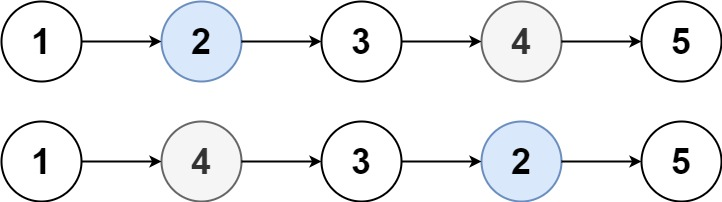# 1721. Swapping Nodes in a Linked List

Swapping Nodes in a Linked List - LeetCode

You are given the head of a linked list, and an integer k. Return __the head of the linked list after swapping the values of the __kth __node from the beginning and the __kth node from the end (the list is 1-indexed).

Example 1:Input: head = [1,2,3,4,5], k = 2 Output: [1,4,3,2,5] Example 2: Input: head = [7,9,6,6,7,8,3,0,9,5], k = 5 Output: [7,9,6,6,8,7,3,0,9,5]

Constraints:

``````The number of nodes in the list is n.
1 <= k <= n <= 105
0 <= Node.val <= 100
``````

• code
``````class Solution:
def swapNodes(self, head: Optional[ListNode], k: int) -> Optional[ListNode]:
length = 0
while l:
length += 1
l = l.next

startk = k
while startk > 0:
first_node = first_node.next
startk -= 1

endk = length - k + 1
while endk > 0:
second_node = second_node.next
endk -= 1

first_node.val, second_node.val = second_node.val, first_node.val

``````
• code one pass
``````class Solution:
def swapNodes(self, head: Optional[ListNode], k: int) -> Optional[ListNode]:
first = second = None
ct = 1

while cur:
if second:
second = second.next
if ct == k:
first = cur